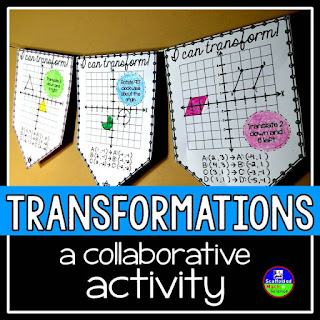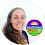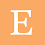## Pages

### How to find inverse functions with a hole punch

Are your algebra students learning how to find inverse functions? Here's how to make the process of finding function inverses easy, visual and hands-on-- with a hole punch! This same process can also be used for reflecting any graph or geometric shape over the x-axis, y-axis, y = x or any other line of symmetry on the coordinate plane.

First, let's do a quick discovery into function inverses using a square coordinate grid, a hole punch and a couple data tables where we'll record our coordinate points:

After punching a few holes over y = x, we start to notice that the x and y coordinates swap places in inverses. Now, let's move on to a linear graph:

Our original graph is y = 2x + 4. Using what we learned from our discovery, when we fold the coordinate grid along y = x and punch holes along y = 2x + 4, we'll get its inverse y = 1/2x - 2. Here's a quick video showing the process with a quadratic:

If our original graph crosses y = x, the points aren't as clear, but the process still works. Here I marked the vertex of each graph:

When we find the inverse of a radical function, we'll find half a quadratic function, or a quadratic with its domain restricted:

And this same process works for reflecting geometric shapes over either axis, y = x or any other line of symmetry on the coordinate plane. Here's an example of a geometric transformation over y = x using a hole punch. I connected the holes using a ruler.

You can grab the pack of grids and graphs I used in this post here in my Google Drive. My hole punch is 1/8th-inch that I had gotten at a craft store years ago, but any hole punch will work as long as it can reach your points.

The hole punch I used in the videos is a 1/8-inch with a 2-inch reach, and looks like the one above. I had originally gotten mine from a craft store, but this screenshot came from Amazon.

An algebra 2 teacher recently requested an inverse functions word wall reference, so I just added this inverse functions reference to my algebra 2 word wall

Related activitiesGeometric transformations math pennant

1.1.2.